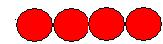Courses

# Test: Three Dimensional Packing

## 10 Questions MCQ Test Chemistry for JEE | Test: Three Dimensional Packing

Description
This mock test of Test: Three Dimensional Packing for JEE helps you for every JEE entrance exam. This contains 10 Multiple Choice Questions for JEE Test: Three Dimensional Packing (mcq) to study with solutions a complete question bank. The solved questions answers in this Test: Three Dimensional Packing quiz give you a good mix of easy questions and tough questions. JEE students definitely take this Test: Three Dimensional Packing exercise for a better result in the exam. You can find other Test: Three Dimensional Packing extra questions, long questions & short questions for JEE on EduRev as well by searching above.
QUESTION: 1

### What is the coordination number in each sphere in the packing as shown in the diagram?Solution:

Linear Arrangement in 1-D paking i.e., the nearest neighbour which toches a particular atom is its coordination no. in the given diagram 2nd atom is toched with 1st nd 3rd atom therefore its coordination no. is 2

QUESTION: 2

### What is the distinguishing layer between hexagonal close packing and cubic close packing?

Solution:

When tetrahedral voids of the second layer  are  covered by the spheres of the third layer. In this case, the spheres of the third layer are exactly aligned with those of the first layer. Thus, the pattern of spheres is repeated in alternate layers. This pattern is often written as ABAB ....... pattern. This  type of packing is called hexagonal close packing (hcp).

When octahedral voids of the second layer  are  covered by the spheres of the third layer. In this case, the spheres of the third layer are are not aligned with those of either the first or the second layer. This arrangement is called -C- type.This pattern of layers is often written as ABCABC ........... This type of packing is called cubic close packing (ccp) or face-centred cubic (fcc) close packing.

QUESTION: 3

### How many atoms form an octahedral void?

Solution:

B type of atoms are at 6 face centres, C type of atoms occupy all tetrahedral voids and D type atoms occupy all octahedral voids.

QUESTION: 4

Which of the following best describes a tetrahedral void?

Solution:

A tetrahedral void is formed when 4 spherical structures are arranged in a cubic form.
As the spheres leave a void between them when they join, the void/ empty space formed is called a tetrahedral void.  And this void is the unoccupied space formed when 4 spheres are put together in a cubic structure.

QUESTION: 5

How many tetrahedral voids will be formed, if 2n Octahedral voids are formed in the cubic close packing?

Solution:

If number of close packed spheres be 'N' , then
Number of Octahedral voids generated = N.
Number of Tetrahedral voids generated = 2N.

So, according to the above explanation, if 2N Octahedral voids are formed, then Tetrahedral voids generated will be = 2(2N) ⇒ 4N.

QUESTION: 6

The constituent particles (atoms/molecules/ions) forming three dimensional structure of solids are assumed to be

Solution:

The constituent particles (atoms/molecules/ions) forming three dimensional structure of solids are assumed to be. These are assumed to be identical hard spheres.

QUESTION: 7

Why it is impossible to pack atoms, molecules and ions without any empty space between them?

Solution:

Close packing in crystals refers to space efficient arrangement of constituent particles in a crystal lattice. To understand this packing more clearly we have to assume all particles (atoms, molecules and ions) are of the same spherical solid shape.

So the unit cell of a lattice is a cubic shape. Now when we stack spheres in the cell, there will always be some empty spaces. To minimize these empty spaces, the arrangement of these spheres must be very efficient. The spheres should be arranged as close together as possible to eliminate empty spaces.

QUESTION: 8

The arrangement of spheres present in two dimensional hexagonal close packing is:

Solution:

In hcp the odd numbered layers are alike and the even numbered layers are alike so the arrangement of spheres is ABAB type.

QUESTION: 9

Na-atom crystallizes and forms a bcc lattice. The edge length (a) = 4.29Ao. What will be the radius of Na-atom?

Solution:

In a body centered cubic lattice, atoms touch along the body diagonal of the cube.Thus

4r=3–√a

or r=3–√4a

=(3–√4)(4.29A°)=1.86A°

QUESTION: 10

How many types of voids are generated if the spheres of second layer are placed in the depressions of a 2D hcp?

Solution:

Two types of voids are generated if the spheres of second layer are placed in the depressions of a 2D hcp – octahedral and tetrahedral void.